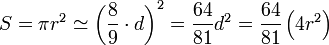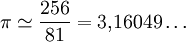# Number Pi

Index

• Summary

• Keywords

• Contents

• Origin of number π and of its name.

• Features of number π.

• The approximations of number π in Ancient Times.

• Mathematics of Ancient Egypt

• Mathematics of Mesopotamia

• Mathematics of Classical Antiquity

• Chinese Mathematics

• Indian Mathematics

• Islamic Mathematics

• Table of approximations

• The approximations of number π in the Modern Times

• Value of π with 100 places.

• Bibliography

• Personal Opinion

Summary

Pi or π is a mathematical constant whose value is the ratio of any circle's circumference respect to its diameter in Euclidean space; this is the same value as the ratio of a circle's area to the square of its radius. π is one of the most important mathematical and physical constants: many formulae from mathematics, science, and engineering involve π. π is an irrational number, which means that its value cannot be expressed exactly as a fraction m/n, where m and n are integers. Consequently, its decimal representation never ends or repeats.

Keywords

• Radius - is any line segment from its center to its perimeter.

• Decimal places - they are numbers followings the point.

• Circumference - is the distance around a closed curve.

Contents

• Origin of number π and of its name.

• History

The earliest evidenced conscious use of an accurate approximation for the length of a circumference with respect to its radius is of 3+1/7 in the designs of the Old Kingdom pyramids in Egypt. The Great Pyramid at Giza, constructed c.2550-2500 BC, was built with a perimeter of 1760 cubits and a height of 280 cubits; the ratio 1760/280 ≈ 2π. Egyptologists such as Professors Flinders Petrie  and I.E.S Edwards have shown that these circular proportions were deliberately chosen for symbolic reasons by the Old Kingdom scribes and architects.

The name

The name of the Greek letter π  is pi, and this spelling is commonly used in typographical contexts when the Greek letter is not available, or its usage could be problematic. It is not capitalised (Π) even at the beginning of a sentence. When referring to this constant, the symbol π is always pronounced /'paɪ/, "pie" inEnglish, which is the conventional English pronunciation of the Greek letter. In Greek, the name of this letter is pronounced [pi].

The constant is named "π" because "π" is the first letter of the Greek words περιφέρεια (periphery) and περίμετρος (perimeter), probably referring to its use in the formula to find the circumference, or perimeter, of a circle.

• Features of number π.

• Definition

Euclid was the first to show that the ratio of a circle to its diameter is a constant quantity. However, there are various definitions of the number π, but the most common is: π is the ratio between the length of a circle to its diameter.

Irrational and transcendental number

π is an irrational number, which means it can not be expressed as a fraction of two integers, as demonstrated by Johann Heinrich Lambert in 1761 (or 1767). It is also a transcendental number, which means it is not the root of any polynomial with integer coefficients. In the nineteenth century German mathematician Ferdinand Lindemann proved this fact and permanently closing the problem of squaring the circle indicating that there isn't solution.

• The approximations of number π in

• The Ancient Times.

• Mathematics of Ancient Egypt

The approximate value of π in ancient Egypt was wrote by scribe Ahmes in 1800 a. C., in the Rhind papyrus, where he used an approximate value of π by saying that: the area of a circle is similar to a square whose side equals the diameter of the circle decreased in 1 / 9, that's equal to 8 / 9 of the diameter. In modern notation:• Mathematics of Mesopotamia

Some mathematicians of of Mesopotamia used in the calculation of segments, values of π equal to 3, reaching in some cases approximate values, such as 3 + 1 / 8.

• Mathematics of Classical Antiquity

The Greek mathematician Archimedes (III century BC) was able to determine the value of π between the interval by 3 10/71, as the minimum value, and 3 1 / 7, the maximum value. With this approximation of Archimedes gets a value with an error of between 0.024% and 0.040% on the actual value. The method used by Arquímedes was simple and consisted circumscribe and inscribe regular polygons of n-sided circles and calculate the perimeter of these polygons. Archimedes started with hexagons circumscribed and inscribed, and was doubling the number of sides to reach 96-sided polygons.

• Chinese Mathematics

In 263, the mathematician Liu Hui was the first to suggest that 3.14 was a good approximation using a polygon of 968 or 1926 sides. Later  he estimated the value of π as: 3,14159 using a polygon of 3,072 sides.
In finals of V century, the Chinese mathematician and astronomer Zu Chongzhi calculated the value of π as: 3.1415926 and which he called "default" and 3.1415927 "excess value" and gave two rational approximations of π: 22 / 7 and 355/113, both well-known, the second one being so good and precise that wassn't equaled until more than nine centuries later, in the XV century.

• Indian Mathematics

Indian mathematician, Aryabhata estimated the value of π as 3.1416 using a regular polygon of 384 sides inscribed. Around 1400 Madhava get an accurate approximation to 11 digits (3.14159265359), being the first to use series to estimate.

• Islamic Mathematics

In the ninth century Al-jwarizmi in his "Algebra" (Hisab al yabr ua al muqabala) notes that the practical man used 22 / 7 as the value of π, the geometer uses 3, and the astronomer 3.1416. In the fifteenth century, the Persian mathematician al-Kashi Ghiyath was able to calculate the approximate value of π with nine digits, using a sexagesimal numerical basis, equivalent to a 16 digit decimal approximation: 2π = 6.2831853071795865.

• Table of approximations

 Year Mathematician or document Culture Approximation Error(parts per million) ~1900 b. C. Papyrus of Ahmes Egyptian 28/34 ~ 3,1605 6016 ppm ~1600 b. C. Tablet of Susa Babylonian 25/8 = 3,125 5282 ppm ~600 b. C. The Bible (Reyes I, 7,23) Jewish 3 45070 ppm ~500 b. C. Bandhayana Indian 3,09 16422 ppm ~250 b. C. Archimedes from Siracusa Greek beetwen 3 10/71 y 3 1/7 he used 211875/67441 ~ 3,14163 <402 ppm 13,45 ppm ~150 Claudius Ptolemy Greek-Egyptian 377/120 = 3,141666... 23,56 ppm 263 Liu Hui Chinese 3,14159 0,84 ppm 263 Wang Fan Chinese 157/50 = 3,14 507 ppm ~300 Chang Hong Chinese 101/2 ~ 3,1623 6584 ppm ~500 Zu Chongzhi Chinese entre 3,1415926 y 3,1415929empleó 355/113 ~ 3,1415929 <0,078 ppm0,085 ppm ~500 Aryabhata Indian 3,1416 2,34 ppm ~600 Brahmagupta Indian 101/2 ~ 3,1623 6584 ppm ~800 Al-Juarismi Persian 3,1416 2,34 ppm 1220 Fibonacci Italian 3,141818 72,73 ppm 1400 Madhava Indian 3,14159265359 0,085 ppm 1424 Al-Kashi Persian 2π = 6,2831853071795865 0,1 ppm

• The approximations of number π in

• The Modern Times

When the first computers were designed, there began to appear programs for calculating the number π with figures as much as possible. In 1949, ENIAC was able to break all records, earning 2037 decimal places in 70 hours, a few years later (1954) a NORAC arrived to 3092 figures. During the decade of the 1960s IBM were breaking records, until a 7030 IBM was able to reach in 1966 to 250,000 decimal places (8 h 23 min). During this time computers were being tested new algorithms for generating sets of numbers from π.

In 2009 they found more than two and a half billion decimals using a supercomputer T2K Tsukuba System, formed by 640 high-performance computers, which together get processing speeds of 95 teraflops. To do this it took 73 hours and 36 minutes.

 Year Discoverer Computer used Number of decimal places 1949 G.W. Reitwiesner and others ENIAC 2.037 1954 NORAC 3.092 1959 Guilloud IBM 704 16.167 1967 CDC 6600 500.000 1973 Guillord y Bouyer CDC 7600 1.001.250 1981 Miyoshi y Kanada FACOM M-200 2.000.036 1982 Guilloud 2.000.050 1986 Bailey CRAY-2 29.360.111 1986 Kanada y Tamura HITAC S-810/20 67.108.839 1987 Kanada, Tamura, Kobo and others NEC SX-2 134.217.700 1988 Kanada y Tamura Hitachi S-820 201.326.000 1989 Brothers Chudnovsky CRAY-2 y IBM-3090/VF 480.000.000 1989 Brothers Chudnovsky IBM 3090 1.011.196.691 1991 Brothers Chudnovsky 2.260.000.000 1994 Brothers Chudnovsky 4.044.000.000 1995 Kanada y Takahashi HITAC S-3800/480 6.442.450.000 1997 Kanada y Takahashi Hitachi SR2201 51.539.600.000 1999 Kanada y Takahashi Hitachi SR8000 68.719.470.000 1999 Kanada y Takahashi Hitachi SR8000 206.158.430.000 2002 Kanada and others Hitachi SR8000/MP 1.241.100.000.000 2004 Hitachi 1.351.100.000.000 2009 Daisuke Takahashi T2K Tsukuba System 2.576.980.370.000

5. Value of π with 100 places.

This is the value of π with its first 100 places:

3, 1415926535 8979323846 2643383279 5028841971 6939937510
5820974944 5923078164 0628620899 8628034825 3421170679

Bibliography

Internet, especially Wikipedia but not at all, I used other websites too.

Personal Opinion

I think this work is very interesting because I could to learn things about very specific number, the number π.

 Enviado por: Karo1 Idioma: inglés País: España

Palabras clave:
Te va a interesar